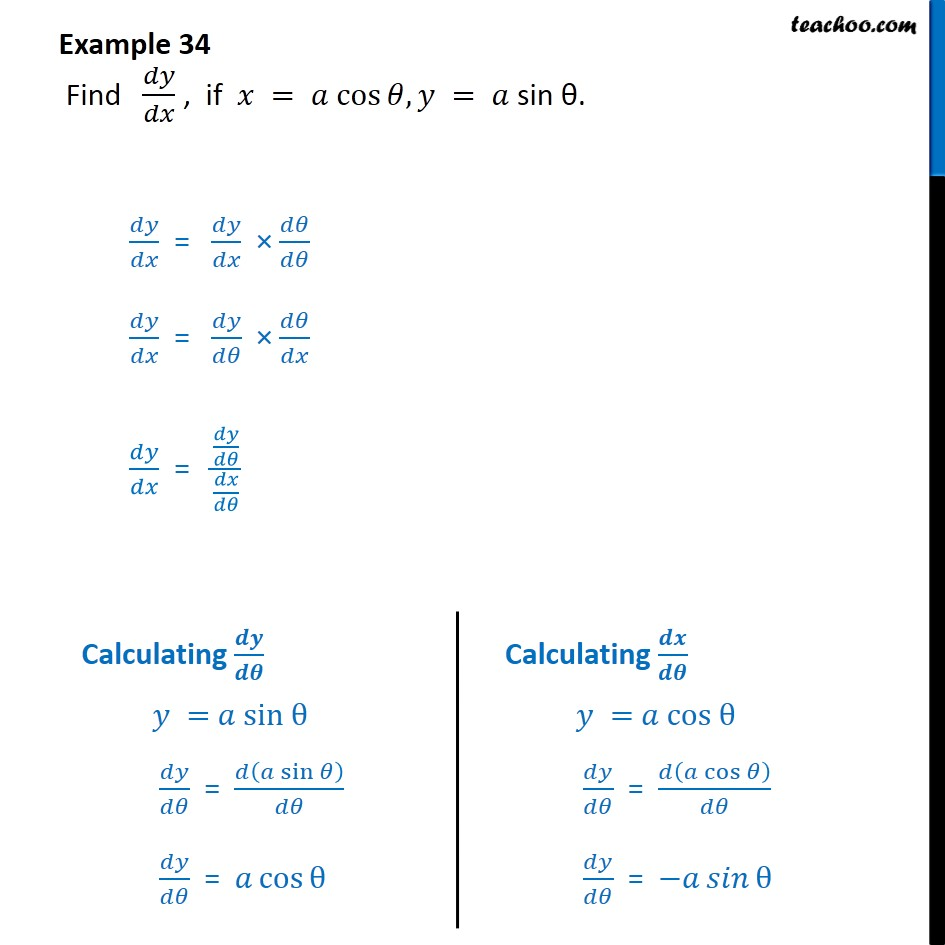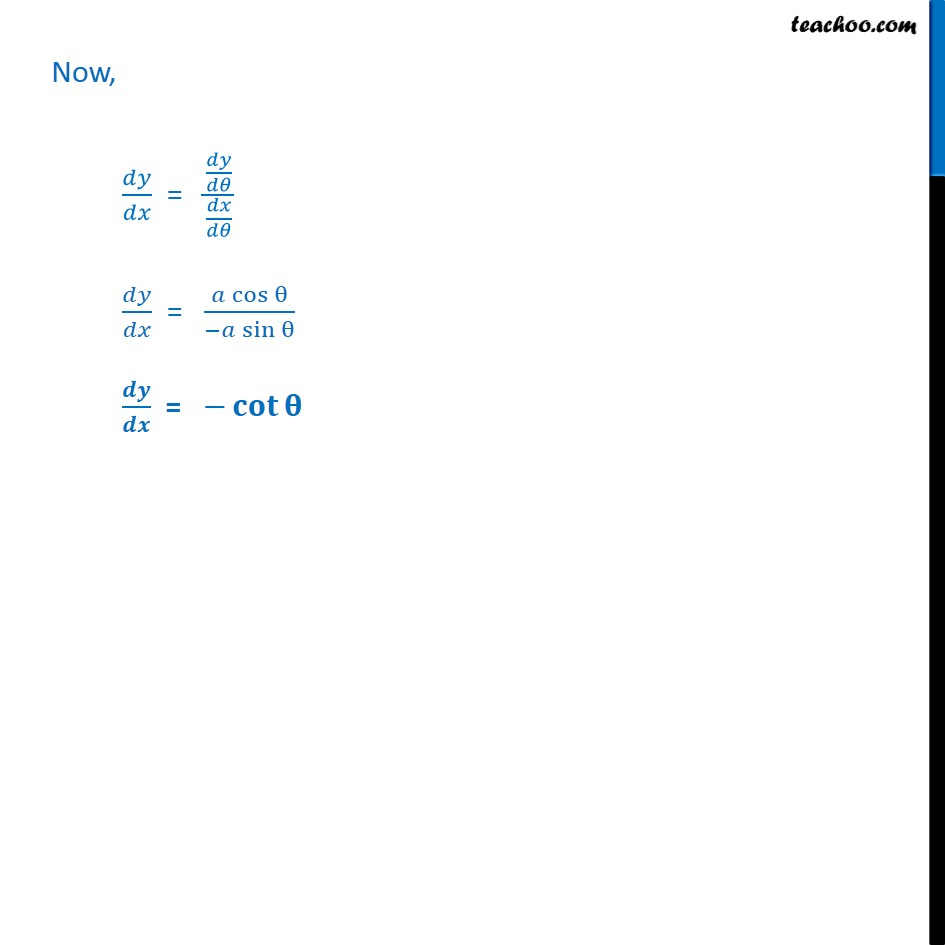1. Chapter 5 Class 12 Continuity and Differentiability
2. Concept wise
3. Derivatives in parametric form

Transcript

Example 34 Find 𝑑𝑦/𝑑𝑥 , if 𝑥 = 𝑎 cos⁡𝜃, 𝑦 = 𝑎 sin θ. 𝑑𝑦/𝑑𝑥 = 𝑑𝑦/𝑑𝑥 × 𝑑𝜃/𝑑𝜃 𝑑𝑦/𝑑𝑥 = 𝑑𝑦/𝑑𝜃 × 𝑑𝜃/𝑑𝑥 𝑑𝑦/𝑑𝑥 = (𝑑𝑦/𝑑𝜃)/(𝑑𝑥/𝑑𝜃) Now, 𝑑𝑦/𝑑𝑥 = (𝑑𝑦/𝑑𝜃)/(𝑑𝑥/𝑑𝜃) 𝑑𝑦/𝑑𝑥 = (𝑎 cos⁡θ)/(−𝑎 sin⁡θ ) 𝒅𝒚/𝒅𝒙 = −𝐜𝐨𝐭⁡𝛉

Derivatives in parametric form